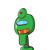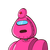# in 3 numbers the mean of the square of first 2 numbers is 26 and the mean of the square of the second and the first is 50 and the

in 3 numbers the mean of the square of first 2 numbers is 26 and the mean of the square of the second and the first is 50 and the mean of the square of the third and the first number is 40 then what are the three numbers? ​

### 2 thoughts on “in 3 numbers the mean of the square of first 2 numbers is 26 and the mean of the square of the second and the first is 50 and the”

1.Let the three numbers be x,y and z

It is given

x^2+y^2=26×2=52….(1)

y^2+z^2=100….(2)

z^2+x^2=80…(3)

from ,(2)

y^2=100-z^2

put this value of y^2 in (1)

x^2+100-z^2=52

x^2-z^2=-48

Add this equation to (3) you will get

2x^2=32 which gives x=4

Put this value of x in equation (1) and (3) you will get y=6 and z=8

So the numbers are 4,6,8

2.